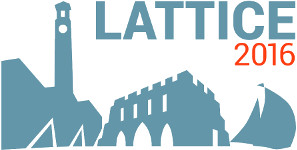#The 34th International Symposium on Lattice Field Theory (Lattice 2016)

Jul 24 – 30, 2016
Highfield Campus, University of Southampton
Europe/London timezone

## Estimating excited-state contamination in nucleon correlators using experimental data

Jul 29, 2016, 2:20 PM
20m
Building B2a Room 2077 (Highfield Campus, University of Southampton)

### Building B2a Room 2077

#### Highfield Campus, University of Southampton

Highfield Campus, Southampton SO17 1BJ, UK

### Speaker

Dr Maxwell Hansen (University of Mainz)

### Description

Excited-state contamination is one of the dominant uncertainties in lattice calculations of nucleon charges and form factors. To estimate this uncertainty one needs the finite-volume energy spectrum as well as the finite-volume matrix elements that determine the coefficients of excited state exponentials. In this work we use experimental data, supplemented by chiral perturbation theory (ChPT), to estimate these quantities. We first use the Lüscher quantization condition, extended to spin-half particles, to calculate finite-volume $N \pi$ states from $N \pi$ scattering phase shifts. We then study the excited state coefficients using an extension of the Lellouch-Lüscher formalism. This gives a relation between finite- and infinite-volume matrix elements. Both matrix elements of the axial current and the nucleon interpolating fields arise. While the former can be constrained experimentally, the latter are generally unknown and depend on the details of the lattice calculation. We examine various scenarios and find that excited-state contamination can be larger than previous ChPT studies suggest.

### Primary author

Dr Maxwell Hansen (University of Mainz)

### Co-author

Dr Harvey Meyer (University of Mainz)

 Slides